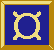# ż Fudget Library Reference Manual ż

Created from the Fudget Library sources on Mon Aug 9 17:27:21 CEST 1999

## GuiElems

Selecting from dynamic lists of alternatives:
``` oldFilePickF :: F String (InputMsg String) ```
Miscellaneous (the rest):
``` oldButtonF :: (Graphic b, Show a, ColorGen a, Graphic c) => Alignment -> Distance -> FontName -> ColorSpec -> a -> [(ModState, KeySym)] -> b -> F c Click ```
``` oldPopupMenuF :: (Graphic c, Eq a) => ColorName -> Bool -> FontName -> Button -> ModState -> [(ModState, KeySym)] -> [(a, b)] -> (a -> c) -> F d e -> F (Either [(a, f)] d) (Either a e) ```

## Combinators

Miscellaneous (the rest):
``` inputListLF :: (Eq a) => Placer -> [(a, InF b c)] -> F [(a, b)] (InputMsg [(a, c)]) ```
``` inputPairLF :: Orientation -> InF a b -> InF c d -> F (a, c) (InputMsg (b, d)) ```

## InfixOps

Miscellaneous (the rest):
``` infixl 9 >#+< ```
``` >#+< :: (F a b, Orientation) -> F c d -> F (Either a c) (Either b d) ```
``` infixl 9 >#==< ```
``` >#==< :: (F a b, Orientation) -> F c a -> F c b ```

## Layout

Miscellaneous (the rest):
``` data Orientation = ... ```
``` compLF :: (F a b, Orientation) -> F c d -> F (Either a c) (Either b d) ```
``` listLF :: (Eq a) => Placer -> [(a, F b c)] -> F (a, b) (a, c) ```
``` serCompLF :: (F a b, Orientation) -> F c a -> F c b ```
``` untaggedListLF :: Placer -> [F a b] -> F (Int, a) b ```

## Containers

Miscellaneous (the rest):
``` oldHscrollF :: Bool -> (Point, Point) -> F a b -> F a b ```
``` oldScrollF :: Bool -> (Point, Point) -> F a b -> F a b ```
``` oldVscrollF :: Bool -> (Point, Point) -> F a b -> F a b ```
``` scrollShellF :: String -> (Point, Point) -> F a b -> F a b ```

## StreamProc

Miscellaneous (the rest):
``` concSP :: SP [a] a ```
``` concmapSP :: (a -> [b]) -> SP a b ```
``` mapstateSP :: (a -> b -> (a, [c])) -> a -> SP b c ```